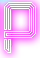# Forest

#### Statement

Bruce Force is standing in the forest. He wonders what is the tree trunk the farthest away which is not blocked from his view by other tree trunks.

Bruce has made a map of the trees in the forest. The map shows his current position as the origin of a cartesian coordinate system. Tree i is shown on the map as a circle with the center (xi, yi) and radius ri. You may assume that a tree trunk is visible if and only if there exists a line segment on the map from the origin (0, 0) to a point on the border of the circle representing the tree trunk, where the line segment does not intersect or touch another circle.

#### Input:

The input contains several test cases. The first line of each test case contains one integer n, where n specifies how many trees are on the map. The following n lines contain 3 integers xi, yi, ri where (xi, yi) is the center of the circle representing tree trunk i, and ri is the radius of the circle. You may assume that no two circles in the input intersect, i.e., for any two circles, the distance between their centers is more than the sum of their radii. Moreover, you may assume that no circle contains the origin. The last test case is followed by a line containing one zero.

Hint: In the second test case, the first four trees block the view of all trees farther away than these four trees.

#### Output:

For each test case, print one line with the maximum euclidean distance from the origin to a visible tree. The distance to a tree should be measured using the point of the tree closest to the origin, no matter if this point is in fact visible or not.

Round the answer to 3 digits after the decimal point.

#### Constraints:

• 1 ≤ n ≤ 1000
• -10000 ≤ xi, yi ≤ 10000
• 1 ≤ ri ≤ 1000

#### Example input:

```3
10 10 11
1 1 1
-20 -10 20
5
1 2 2
-2 1 1
2 -1 1
-1 -2 2
10000 -10000 1000
0
```

```3.142
1.236
```

#### Time and memory limit:

• 1 second
• 64MB

Problem source: URI